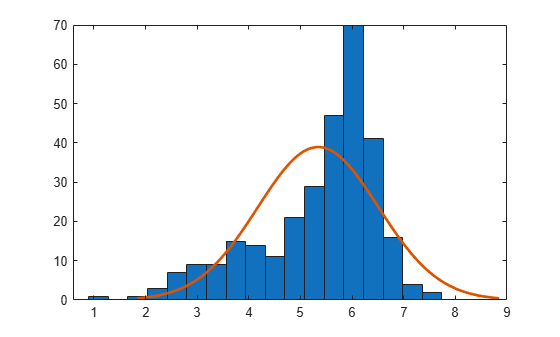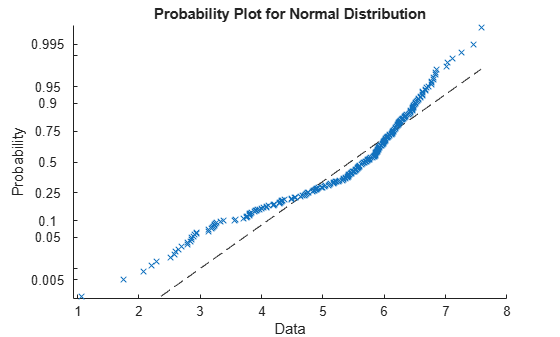# Exploratory Analysis of Data

This example shows how to explore the distribution of data using descriptive statistics.

### Generate sample data

Generate a vector containing randomly-generated sample data.

```rng default % For reproducibility x = [normrnd(4,1,1,100),normrnd(6,0.5,1,200)];```

### Plot a histogram

Plot a histogram of the sample data with a normal density fit. This provides a visual comparison of the sample data and a normal distribution fitted to the data.

`histfit(x)`The distribution of the data appears to be left skewed. A normal distribution does not look like a good fit for this sample data.

### Obtain a normal probability plot

Obtain a normal probability plot. This plot provides another way to visually compare the sample data to a normal distribution fitted to the data.

`probplot('normal',x)`The probability plot also shows the deviation of data from normality.

### Create a box plot

Create a box plot to visualize the statistics.

`boxplot(x)`The box plot shows the 0.25, 0.5, and 0.75 quantiles. The long lower tail and plus signs show the lack of symmetry in the sample data values.

### Compute descriptive statistics

Compute the mean and median of the data.

`y = [mean(x),median(x)]`
```y = 1×2 5.3438 5.6872 ```

The mean and median values seem close to each other, but a mean smaller than the median usually indicates that the data is left skewed.

Compute the skewness and kurtosis of the data.

`y = [skewness(x),kurtosis(x)]`
```y = 1×2 -1.0417 3.5895 ```

A negative skewness value means the data is left skewed. The data has a larger peakedness than a normal distribution because the kurtosis value is greater than 3.

### Compute z-scores

Identify possible outliers by computing the z-scores and finding the values that are greater than 3 or less than -3.

```Z = zscore(x); find(abs(Z)>3);```

Based on the z-scores, the 3rd and 35th observations might be outliers.